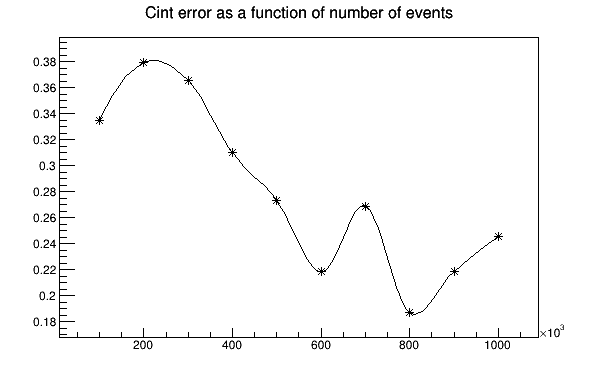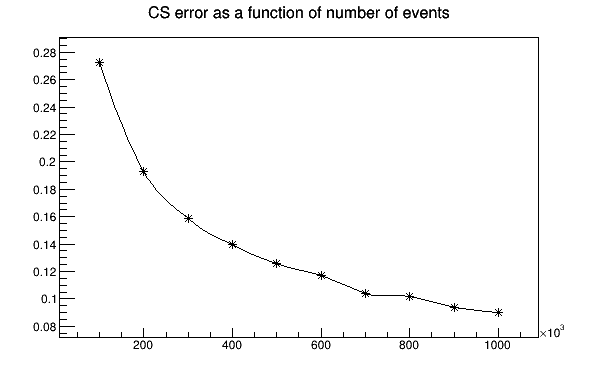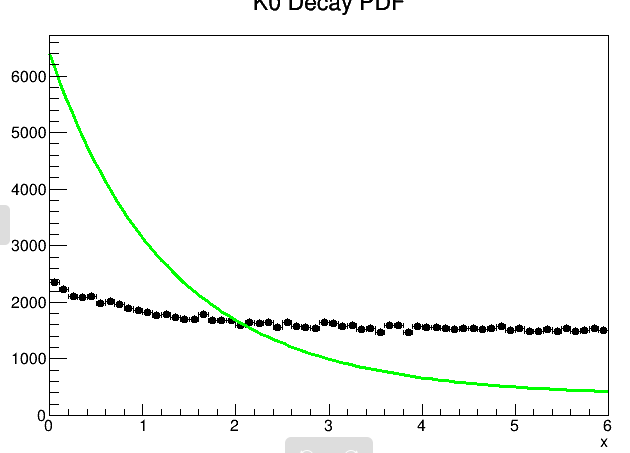# Improving error on a roofit with correlated coefficient

Hello,
I’m trying to generate a toy model and fit the parameters Cs,Cint on it with a pdf of the following form :
f(t)=CSexp(-x) +CLexp(-xTau1/Tau2)+Cint cos(phi-mx)exp(-xTau1/Tau3)
Tau2 >>Tau1 and Tau3=2
Tau1
Physically, the last term is coming from interferance between other two channels.
Typical value of the parameters are CS=0.43, Cint=0.12*0.28 , CL=1 ( I don’t fit it, it is just to normalize other coefficients)

First try building the pdf with string expression :

``````    RooRealVar x("x", "x", startt, endd);
RooRealVar CS("CS", "CS", CSv, 0.00, 1.0);
RooRealVar Cint("Cint", "Cint", Cintv, 0.00, 1.0);

ostringstream decayform, decayfit;

decayform<<CL<<"*exp(-x*"<<tauKS/tauKL<<") + CS*exp(-x*"<<tauKS/tauKS<<") + 2*exp(-x*"<<tauKS/tauK<<")*"<<DiluCon<<"*Cint*cos("<<Phi0<<"-("<<dMK<<"*x/"<<tauKS<<"e-9))";
decayfit<<CL<<"*exp(-x*"<<tauKS/tauKL<<") + CS*exp(-x*"<<tauKS/tauKS<<") + 2*exp(-x*"<<tauKS/tauK<<")*"<<DiluCon<<"*Cint*cos("<<Phi0<<"-("<<dMK<<"*x/"<<tauKS<<"e-9))";
RooGenericPdf decaypdf("decaypdf", "decaypdf", decayform.str().c_str(), RooArgSet(x, CS, Cint));
RooGenericPdf decaypdfit("decaypdfit", "decaypdfit", decayfit.str().c_str(), RooArgSet(x, CS, Cint));

CS.setVal(CSv);
Cint.setVal(Cintv);

RooDataSet *data = decaypdf.generate(x, events);

TH1 *roohist = data->createHistogram("roohist", x, Binning(bins,startt,endd));

RooDataHist rdh("rdh", "rdh", x, roohist);

CS.setVal(0);
Cint.setVal(0);

std::unique_ptr<RooFitResult> fitResult{decaypdfit.fitTo(rdh, Save())};

``````

With this code I found really bad error on Cint : around 40 %

With another form using Rooaddpdf and then normalising the coefficient (dividing them by 1+CS+Cint), the fit now gives Cint with an error of 15%.
The code is :

``````ostringstream decayKS,decayKL,decayKint;

decayKS<<"exp(-x*"<<tauKS/tauKS<<")";
decayKL<<"exp(-x*"<<tauKS/tauKL<<")";
decayKint<<"2*exp(-x*"<<tauKS/tauK<<")*"<<DiluCon<<"*cos(Phi0-("<<dMK<<"*x/"<<tauKS<<"e-9))";

RooRealVar x("x", "x", startt, endd);
RooRealVar CS("CS", "CS", CSv/(1+CSv+Cintv*DiluCon), 0.00, 1.0);
RooRealVar Cint("Cint", "Cint", Cintv/(1+CSv+Cintv*DiluCon), 0.00, 1.0);
RooRealVar Phi0("Phi0", "Phi0", gRandom->Gaus(Phi0v,0.1), -1, 1);
RooGaussian Phicon("Phicon","Phicon", Phi0, RooConst(Phi0v), RooConst(0.1/*From Precision Measurement Paper*/)) ;

RooGenericPdf decaypdfKS("decaypdfKS", "decaypdfKS", decayKS.str().c_str(), RooArgSet(x,Phi0));
RooGenericPdf decaypdfKL("decaypdfKL", "decaypdfKL", decayKL.str().c_str(), RooArgSet(x,Phi0));
RooGenericPdf decaypdfKint("decaypdfKint", "decaypdfKint", decayKint.str().c_str(), RooArgSet(x,Phi0));

``````

This is done with phi fixed or constrained around 0.20 for 100k events in total, I end up at best with a 15% error on Cint which is way more than 1/sqrt(Nint).
I don’t understand where the difference over the result between two techniques come from since I use the same number of event and the same fitting technique, I just use two different way to build the pdf isn’t it ?
Also I noticed that CS and Cint are really strongly correlated ( correlation of -0.96 on average !) which may explain why the fit is not that good. Do you have any idea of how to improve it ?

Moreover, I would like to do the same with destructive interferences ( phi close to pi ) but for that rooaddpdf no longer works because the part of the pdf with interference has now negative value.

Hi @hjacinto; I’m inviting @jonas, our RooFit expert, to the topic. I’m sure he can help you with this.

Cheers,
J.

Hi @hjacinto, welcome to the ROOT forum!

Three things you need to know to understand the problem:

1. This does not work like you intended:

``````    decayform<<CL<< ...
``````

When you write a RooRealVar to an output stream, it will put the numerical value and not the name. So RooFit has no idea this is the CL parameter and just treats it at a hardcoded number. You have this problem in other places in the code too.

2. The RooAddPdf gives you different results because it first normalizes all of the components, which is not what you want for amplitudes. You should represent your amplitudes with RooFormulaVar and not RooGenericPdf so they don’t normalize themselves, and then use RooRealSumPdf instead of RooAddPdf. A RooRealSumPdf only normalizes itself after adding the components and doesn’t normalize the components before.

3. Goes in the same direction as 2: If you use the RooRealSumPdf, you can also consider the negative amplitudes. Different from the RooAddPdf, the RooRealSumPdf only requires that the sum is always positive, not the components.

I hope this helps to clear your inconsistencies!

Cheers,
Jonas

Hello Jonas,
Thanks a lot this helps me a lot!
I will modify my code and let you if I face any issue afterwards!
Best,
Hugo

Thanks a lot for correcting my mistakes !
The result are still not that good I have a quite big error on my fitted coefficient (error that goes even 2-3 times bigger with negative interferences), here is my complete code for the fit

`````` //ROOFit Parameter Estimation - {Cs,Cint,Phi0} with Recursive Optimization
#include <TH1F.h>
#include <TH2F.h>
#include <TCanvas.h>
#include <TFile.h>
#include <TTree.h>
#include <THStack.h>
#include <TVector3.h>
#include <TF1.h>
#include <RooMCStudy.h>

//ROOFit Parameter Estimation - {Cs,Cint,Phi0} with Binned Data

void roof_CiPh2(int bins, int noh, double startt, double endd, int events, double DiluCon ,double CL , double Cintv ,double CSv, double Phi0v)
{
using namespace RooFit;

double tauKS = 0.08954, tauKL = 51.16; //in nano-seconds
double MK = 497.614 /*in MeV/c^2*/,  dMK = 2.1890e-11 /*in MeV/c^2*/;
double tauK = 2.0/((1.0/tauKS)+(1.0/tauKL));

ostringstream decayKS,decayKL,decayKint;

decayKS<<"exp(-x*"<<tauKS/tauKS<<")";
decayKL<<"exp(-x*"<<tauKS/tauKL<<")";
decayKint<<"2*exp(-x*"<<tauKS/tauK<<")*"<<DiluCon<<"*cos(Phi0-("<<dMK<<"*x/"<<tauKS<<"e-9))";

RooRealVar x("x", "x", startt, endd);
RooRealVar CS("CS", "CS", CSv/(1+CSv+Cintv*DiluCon), 0.00, 1.0);
RooRealVar Cint("Cint", "Cint", Cintv/(1+CSv+Cintv*DiluCon), 0.00, 1.0);
RooRealVar Phi0("Phi0", "Phi0", gRandom->Gaus(Phi0v,0.1), -3.14, 3.14);
RooGaussian Phicon("Phicon","Phicon", Phi0, RooConst(Phi0v), RooConst(0.1/*From Precision Measurement Paper*/)) ;

RooFormulaVar decaypdfKS("decaypdfKS", "decaypdfKS", decayKS.str().c_str(), RooArgSet(x,Phi0));
RooFormulaVar decaypdfKL("decaypdfKL", "decaypdfKL", decayKL.str().c_str(), RooArgSet(x,Phi0));
RooFormulaVar decaypdfKint("decaypdfKint", "decaypdfKint", decayKint.str().c_str(), RooArgSet(x,Phi0));
RooRealSumPdf model("model","model",RooArgList(decaypdfKS,decaypdfKint,decaypdfKL),
RooArgList(CS,Cint)) ;
ROOT::Math::MinimizerOptions::SetDefaultMinimizer("Minuit2");

int i=0, j=0;

TH1D CShist("CShist","Parameter Estimation: C_{S};C_{S};Count",100,-0.5,1.5);
TH1D Cinthist("Cinthist","Parameter Estimation: C_{int};C_{int};Count",100,-0.5,1.5);
TH1D CSerhist("CSerhist","Parameter Error: C_{S};Error(C_{S});Count",100,-1,2);
TH1D Cinterhist("Cinterhist","Parameter Error: C_{int};Error(C_{int});Count",100,-1,2);
TH1D Phi0hist("Phi0hist","Parameter Estimation: #phi_{0};#phi_{0};Count",100,-3.14,3.14);//-1.6,1.6);

double corravg = 0;
for(i=0;i<noh;i++)
{
CS.setVal(CSv/(1+CSv+Cintv*DiluCon));
Cint.setVal(Cintv/(1+CSv+Cintv*DiluCon));
Phi0.setVal(gRandom->Gaus(Phi0v,0.1));

RooDataSet *data = model.generate(x, events);

TH1 *roohist = data->createHistogram("roohist", x, Binning(bins,startt,endd));

RooDataHist rdh("rdh", "rdh", x, roohist);

CS.setVal(0.0);
Cint.setVal(0.0);

std::unique_ptr<RooFitResult> fitResult{model.fitTo(rdh, IntegrateBins(0.),ExternalConstraints(Phicon),Save())};

CShist.Fill(CS.getValV()/(1-CS.getValV()-Cint.getValV()*DiluCon));
Cinthist.Fill(Cint.getValV()/(1-CS.getValV()-Cint.getValV()*DiluCon));
CSerhist.Fill(CS.getValV()/(1-CS.getValV()-Cint.getValV()*DiluCon)*sqrt(pow((CS.getError()/CS.getValV()),2)+pow(((CS.getError()+Cint.getError())/(CS.getValV()+Cint.getValV())),2)));
Cinterhist.Fill(Cint.getValV()/(1-CS.getValV()-Cint.getValV()*DiluCon)*sqrt(pow((Cint.getError()/Cint.getValV()),2)+pow(((CS.getError()+Cint.getError())/(CS.getValV()+Cint.getValV())),2)));
Phi0hist.Fill((Phi0.getValV()));
corravg += (fitResult->correlation("CS","Cint")/double(noh));
if(i==(noh-1))
{
RooPlot *xframe = x.frame(Title("K0 Decay PDF"));
rdh.plotOn(xframe);
model.plotOn(xframe,LineColor(kGreen));
xframe->Draw();
}

}

cout<<"AvgCorr{CS,Cint} = "<<corravg<<endl;

cout<<"\n\n";
gStyle->SetOptStat(1111111);
CShist.Draw();
Cinthist.Draw();

CSerhist.Draw();
Cinterhist.Draw();
Phi0hist.Draw();

}

void test4()
{
int bins = 60, noh = 10, events = (300000);
double startt = 0, endd = 6;
double DiluCon = 0.285714286;   //Dilucon = 2.0/7.0;  N(K0)/N(K0bar) = 1.8
double Cint = 0.12;//0.12;
double Phi0 = 0.201357921;//2.94023; //
double CS = 0.43;
double CL = 1;

roof_CiPh2(bins, noh, startt, endd, events, DiluCon,CL, Cint,CS, Phi0);
}

``````

and here is two plots of how the relative error scale with number of total eventsI’m a bit disappointed, it looks like It’s not possible to have reasonable error (below 5%) on Cint with something under 100k event which is what I was hoping for …

Hi, I’m grad that it works better now!

Technically you are doing nothing wrong. Your RooRealSumPdf just has components that are too correlated, in particular the KInt and KL components.

If you `Print()` the RooFitResult after `fitTo()`, you will see that the fit didn’t even converge properly:

``````  RooFitResult: minimized FCN value: 535428, estimated distance to minimum: 0.000149592
covariance matrix quality: Full, accurate covariance matrix
Status : MINIMIZE=1 HESSE=1

Floating Parameter    FinalValue +/-  Error
--------------------  --------------------------
CS    2.8884e-01 +/-  2.26e-02
Cint    8.8927e-02 +/-  2.93e-02
Phi0    1.9851e-01 +/-  1.00e-01
``````

I think I figured out the problem though. Look at how you’re defining the RooRealSumPdf:

``````    RooRealSumPdf model("model","model",
RooArgList(decaypdfKS,decaypdfKint,decaypdfKL),
RooArgList(CS,Cint)) ;
``````

You are having three components, but missing one coefficient. In this case, the third coefficient will be chosen such that the total pdf is normalized, which probably doesn’t even make physically sense because the interference term is not some correction term that makes the amplitude normalized (the pdf should be normalized only after summing the amplitudes). And I guess introduces some bad correlation that prevents the fit from conversion properly.

Should the coefficient for the interference not be something like `sqrt(CS * Cint)` (or another combination of the two)?

``````    RooFormulaVar coef3{"coef3", "sqrt(CS * Cint)", {CS, Cint}};
RooRealSumPdf model("model","model",
RooArgList(decaypdfKS,decaypdfKint,decaypdfKL),
RooArgList(CS,Cint, coef3)) ;
``````

Thanks a lot for your answer, what I was aiming for with those two coefficient is to have something of the form f(t) = C1 fs(t) +C2 fint(t) +(1-C1-C2) fL(t) instead of f(t)=CSfs(t)+Cint fint(t) +CL fL(t) because I thought that both were equivalent by dividing by (1+CS+Cint) which is why I have to propagate the error at the end of my code and to do some algebra to recover the original CS and Cint. Originally I did that to be able to use RooAddPdf that works only when coefficients sum up to 1 (does it?) but I will now use the original form of f(t) since it looks that RooRealSumPdf can deal with unnormalized coefficient ! I will let you know about the outcome !

But from what I know about the physics of the process I’m studying, Cint Cs and CL are not simple functions of one another but the value I’m using are some prevision of the Standard Model given in a paper.

That’s correct, the RooAddPdf would have done `1-C1-C2` indeedBut choosing C3 such that the whole sum is normalized without an additional normalization coefficient is different from that. Sorry for the confusion! But to RooFits defense, it is explained in the documentationhttps://root.cern.ch/doc/master/classRooRealSumPdf.html

1 Like

I’m a bit confused about the documentation of RooRealSumPdf, if I add a third component am i supposed to set extended=true as said in the documentation ? (Quote :" If an coefficient is provided, the PDF can be used as an extended PDF, i.e. the total number of events will be measured in addition to the fractions of the various functions. **This requires setting the last argument of the constructor to ‘true’.")
Because when I do that, the fit goes really bad (it is not even fitting i’s just setting CS Cint to their maximum allowed value so I think there is normalization issue):When I don’t set extended=true but just add a third coefficient (just as you did in your advice), it fits but I still got a 80% error on Cint :

Nono, you can add a third coefficient without doing the extended fit! The documentation is just saying that if you provide n coefficients, you can do an extended fit if you want.

In an extended fit, the integral of the unnormalized RooRealSumPdf is also interpreted as the expected number of events in an additional Poisson term that constrains the expected number of events.

That’s why coefficients are growing so much in the fit, because you observe many events and the RooRealSumPdf needs to be scaled up to match this. So if you want to do an extended fit, the interpretation of the coefficients is a bit different. In particular, you have to adjust their limits accordingly.

Oh ok I see, I think I don’t need to do an extended fit then thanks ! I will see how I can improve the error

I think I’ve done things properly in this last version of the code :

`````` //ROOFit Parameter Estimation - {Cs,Cint,Phi0} with Recursive Optimization
#include <TH1F.h>
#include <TH2F.h>
#include <TCanvas.h>
#include <TFile.h>
#include <TTree.h>
#include <THStack.h>
#include <TVector3.h>
#include <TF1.h>
#include <RooMCStudy.h>
#include "RooRealVar.h"
#include "RooDataSet.h"
#include "RooGaussian.h"
#include "RooConstVar.h"
#include "RooTruthModel.h"
#include "RooFormulaVar.h"
#include "RooRealSumPdf.h"
#include "RooPolyVar.h"
#include "RooProduct.h"
#include "TH1.h"
#include "TCanvas.h"
#include "TAxis.h"
#include "RooPlot.h"

//ROOFit Parameter Estimation - {Cs,Cint,Phi0} with Binned Data

void roof_CiPh2(int bins, int noh, double startt, double endd, int events, double DiluCon ,double CLv , double Cintv ,double CSv, double Phi0v)
{
using namespace RooFit;

double tauKS = 0.08954, tauKL = 51.16; //in nano-seconds
double MK = 497.614 /*in MeV/c^2*/,  dMK = 2.1890e-11 /*in MeV/c^2*/;
double tauK = 2.0/((1.0/tauKS)+(1.0/tauKL));

ostringstream decayKS,decayKL,decayKint;

decayKS<<"exp(-x*"<<tauKS/tauKS<<")";
decayKL<<"exp(-x*"<<tauKS/tauKL<<")";
decayKint<<"2*exp(-x*"<<tauKS/tauK<<")*"<<DiluCon<<"*cos(Phi0-("<<dMK<<"*x/"<<tauKS<<"e-9))";

RooRealVar x("x", "x", startt, endd);
RooRealVar CS("CS", "CS", CSv, 0.00, 1);
RooRealVar CL("CL", "CL", CLv, 0.00, 2);
RooRealVar Cint("Cint", "Cint", Cintv, 0.00, 1);
RooRealVar Phi0("Phi0", "Phi0", gRandom->Gaus(Phi0v,0.1), -3.14, 3.14);
RooGaussian Phicon("Phicon","Phicon", Phi0, RooConst(Phi0v), RooConst(0.1/*From Precision Measurement Paper*/)) ;

RooFormulaVar decaypdfKS("decaypdfKS", "decaypdfKS", decayKS.str().c_str(), RooArgSet(x,Phi0));
RooFormulaVar decaypdfKL("decaypdfKL", "decaypdfKL", decayKL.str().c_str(), RooArgSet(x,Phi0));
RooFormulaVar decaypdfKint("decaypdfKint", "decaypdfKint", decayKint.str().c_str(), RooArgSet(x,Phi0));
RooRealSumPdf model("model","model",RooArgList(decaypdfKS,decaypdfKint,decaypdfKL),
RooArgList(CS,Cint,CL)) ;

int i=0, j=0;
TH1D CLhist("CLhist","Parameter Estimation: C_{L};C_{L};Count",100,-0.5,2);
TH1D CShist("CShist","Parameter Estimation: C_{S};C_{S};Count",100,-0.5,1.5);
TH1D Cinthist("Cinthist","Parameter Estimation: C_{int};C_{int};Count",100,-0.5,1.5);
TH1D CSerhist("CSerhist","Parameter Error: C_{S};Error(C_{S});Count",100,-1,2);
TH1D Cinterhist("Cinterhist","Parameter Error: C_{int};Error(C_{int});Count",100,-1,2);
TH1D Phi0hist("Phi0hist","Parameter Estimation: #phi_{0};#phi_{0};Count",100,-3.14,3.14);//-1.6,1.6);

double corravg = 0;
for(i=0;i<noh;i++)
{
CS.setVal(CSv);
Cint.setVal(Cintv);
CL.setVal(CLv);

Phi0.setVal(gRandom->Gaus(Phi0v,0.1));
CL.setConstant(true);

RooDataSet *data = model.generate(x, events);

TH1 *roohist = data->createHistogram("roohist", x, Binning(bins,startt,endd));

RooDataHist rdh("rdh", "rdh", x, roohist);

CS.setVal(0.0);
Cint.setVal(0.0);

std::unique_ptr<RooFitResult> fitResult{model.fitTo(rdh, IntegrateBins(0.),ExternalConstraints(Phicon),Save())};

CShist.Fill(CS.getValV());
CLhist.Fill(CL.getValV());
Cinthist.Fill(Cint.getValV());
CSerhist.Fill(CS.getError());
Cinterhist.Fill(Cint.getError());
Phi0hist.Fill((Phi0.getValV()));
corravg += (fitResult->correlation("CS","Cint")/double(noh));
if(i==(noh-1))
{
RooPlot *xframe = x.frame(Title("K0 Decay PDF"));
rdh.plotOn(xframe);
model.plotOn(xframe,LineColor(kGreen));
xframe->Draw();
}

}

cout<<"AvgCorr{CS,Cint} = "<<corravg<<endl;

cout<<"\n\n";
gStyle->SetOptStat(1111111);
CShist.Draw();
Cinthist.Draw();
CLhist.Draw();
CSerhist.Draw();
Cinterhist.Draw();
Phi0hist.Draw();

}

void test4()
{
int bins = 60, noh = 100, events = (100000);
double startt = 0, endd = 6;
double DiluCon = 0.285714286;   //Dilucon = 2.0/7.0;  N(K0)/N(K0bar) = 1.8
double Cint = 0.12;//0.12;
double Phi0 = 0.201357921;//2.94023; //
double CS = 0.43;
double CL = 1;

roof_CiPh2(bins, noh, startt, endd, events, DiluCon,CL, Cint,CS, Phi0);
}

``````

However I got inconsistent value and error on Cint at the end and 10% on CS for 100K total event. I begin to feel like it is just not possible to fit those coefficients with decent error on 100k event on Roofit … The fit looks nice when I plot it but when I plot the histogram of errors on coefficient for each fit we can see several “peaks”, maybe there is a local minima somewhere.

This topic was automatically closed 14 days after the last reply. New replies are no longer allowed.